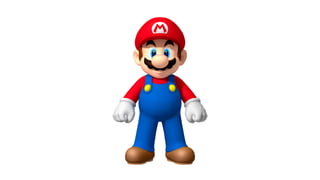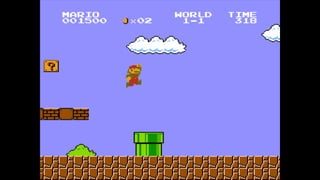Successfully reported this slideshow.

# Graphics Programming for Web Developers×

1 of 77 Ad
1 of 77 Ad

# Graphics Programming for Web Developers

Talk given at Mozilla's first View Source Conference in Portland, 2015. Details out the parallels between graphics and game developments compared to traditional web development.

Talk given at Mozilla's first View Source Conference in Portland, 2015. Details out the parallels between graphics and game developments compared to traditional web development.

### Graphics Programming for Web Developers

1. 1. Graphics for Web Devs Jarrod Overson - Shape Security @jsoverson
2. 2. Yes, we're not game developers. But what are you looking for in your applications?
3. 3. • 60 FPS • Beautiful graphics • Responsive Interaction • Smooth animations
4. 4. When I say "video games" think: "performance critical graphic applications"
5. 5. The web is one of the only computer platforms that hasn't grown with video games pushing the edge. Why?
6. 6. 1996
7. 7. The web may not need games. But losing that discipline has handicapped us.
8. 8. So where do we start? <canvas>
9. 9. document.createElement('canvas'); <canvas> or
10. 10. canvas.width = 1280; canvas.height = 720;
11. 11. var context = canvas.getContext('2d'); or "webgl"
12. 12. context.fillStyle = 'MediumAquaMarine'; context.fillRect(10, 10, 100, 100);
13. 13. for (var i = 0; i < 500; i++) { context.fillRect(10 + i, 10 + i, 100, 100); }
14. 14. for (var i = 0; i < 500; i++) { context.clearRect(0, 0, canvas.width, canvas.height); context.fillRect(10 + i, 10 + i, 100, 100); }
15. 15. setTimeout(draw, 1000/60) requestAnimationFrame(draw)
16. 16. var i = 0; function draw() { if (i < 500) i++; context.clearRect(0, 0, canvas.width, canvas.height); context.fillRect(i + 10, i + 10, 100, 100); requestAnimationFrame(draw); } draw();
17. 17. var i = 0; function draw() { if (i < 500) i++; context.clearRect(0, 0, canvas.width, canvas.height); context.fillRect(i + 10, i + 10, 100, 100); requestAnimationFrame(draw); } draw(); First we update our state.
18. 18. var i = 0; function draw() { if (i < 500) i++; context.clearRect(0, 0, canvas.width, canvas.height); context.fillRect(i + 10, i + 10, 100, 100); requestAnimationFrame(draw); } draw(); Then we render our current state.
19. 19. var i = 0; function draw() { if (i < 500) i++; context.clearRect(0, 0, canvas.width, canvas.height); context.fillRect(i + 10, i + 10, 100, 100); requestAnimationFrame(draw); } draw(); And we do it all over again.
20. 20. Update Render Our loop
21. 21. function loop() { update(); render(); requestAnimationFrame(loop); } loop();
22. 22. Particle System Particles Emitters
23. 23. class ParticleSystem { constructor() { this.particles = []; this.emitters = []; } }
24. 24. class Particle { constructor(position, velocity, acceleration) { this.position = position; this.velocity = velocity; this.acceleration = acceleration; } }
25. 25. class Particle { constructor(position, velocity, acceleration) { this.position = position || {x: 0, y: 0}; this.velocity = velocity || {x: 0, y: 0}; this.acceleration = acceleration || {x: 0, y: 0}; } }
26. 26. class Vector { constructor(x, y) { this.x = x || 0; this.y = y || 0; } }
27. 27. class Particle { constructor(position, velocity, acceleration) { this.position = position || new Vector(0, 0); this.velocity = velocity || new Vector(0, 0); this.acceleration = acceleration || new Vector(0, 0); } }
28. 28. class Emitter { constructor(point, velocity) { this.position = point || new Vector(0, 0); this.velocity = velocity || new Vector(0, 0); } }
29. 29. class ParticleSystem { constructor(width, height) { /* …snipped… */ } render(context) { context.clearRect(0, 0, this.width, this.height); this.emitters.forEach(emitter => emitter.render(context)); this.particles.forEach(particle => particle.render(context)); } }
30. 30. class Particle { constructor(position, velocity, acceleration) { /* … */ } render(context) { context.fillStyle = 'red'; context.fillRect(this.position.x, this.position.y, 2, 2); } }
31. 31. var system = new ParticleSystem(width, height); system.emitters.push(new Emitter(new Vector(10, 10))); system.particles.push(new Particle(new Vector(20, 20))); system.particles.push(new Particle(new Vector(10, 20))); system.particles.push(new Particle(new Vector(20, 10))); function loop() { system.render(context); window.requestAnimationFrame(loop); } loop();
32. 32. var system = new ParticleSystem(width, height); system.addEmitter(320, 180); function loop() { system.update(); system.render(context); window.requestAnimationFrame(loop); } loop();
33. 33. class Emitter { constructor(position, velocity) { /* … */ } render(context) { /* … */ } emitParticle() { return new Particle(this.position.clone(), this.velocity.clone()); } }
34. 34. class Particle { constructor(position, velocity, acceleration) { /* … */ } render(context) { /* … */ } update() { this.velocity.add(this.acceleration); this.position.add(this.velocity); } }
35. 35. class ParticleSystem { constructor(width, height) { /* … */ } addEmitter(x, y, velX, velY) { /* … */ } update() { this.emitters.forEach( emitter => this.particles.push(emitter.emitParticle()) ); this.particles = this.particles.filter(particle => { particle.update(); let pos = particle.position; let outOfBounds = pos.x > this.width || pos.y > this.height || pos.x < 0 || pos.y < 0 return !outOfBounds; }) } }
36. 36. emitParticle() { // Define a maximum angle range in radians. let spread = Math.PI / 32; // Use an angle randomized over a spread so we have more of a "spray" var angle = this.velocity.rad() + spread - (Math.random() * spread * 2); // The magnitude of the emitter's velocity var magnitude = this.velocity.length(); // New velocity based off of the calculated angle and magnitude var velocity = Vector.fromAngle(angle, magnitude); // return our new Particle return new Particle(this.position.clone(), velocity); }
37. 37. emitParticle() { // Define a maximum angle range in radians. let spread = Math.PI / 32; // Use an angle randomized over a spread so we have more of a "spray" var angle = this.velocity.rad() + spread - (Math.random() * spread * 2); // The magnitude of the emitter's velocity var magnitude = this.velocity.length(); // New velocity based off of the calculated angle and magnitude var velocity = Vector.fromAngle(angle, magnitude); // return our new Particle return new Particle(this.position.clone(), velocity); }
38. 38. emitParticle() { // Define a maximum angle range in radians. let spread = Math.PI / 32; // Use an angle randomized over a spread so we have more of a "spray" var angle = this.velocity.rad() + spread - (Math.random() * spread * 2); // The magnitude of the emitter's velocity var magnitude = this.velocity.length(); // New velocity based off of the calculated angle and magnitude var velocity = Vector.fromAngle(angle, magnitude); // return our new Particle return new Particle(this.position.clone(), velocity); }
39. 39. Velocity
40. 40. + Spread
41. 41. - (2 * spread)
42. 42. - (2 * spread) * rand
43. 43. emitParticle() { // Define a maximum angle range in radians. let spread = Math.PI / 32; // Use an angle randomized over a spread so we have more of a "spray" var angle = this.velocity.rad() + spread - (Math.random() * spread * 2); // The magnitude of the emitter's velocity var magnitude = this.velocity.length(); // New velocity based off of the calculated angle and magnitude var velocity = Vector.fromAngle(angle, magnitude); // return our new Particle return new Particle(this.position.clone(), velocity); }
44. 44. emitParticle() { // Define a maximum angle range in radians. let spread = Math.PI / 32; // Use an angle randomized over a spread so we have more of a "spray" var angle = this.velocity.rad() + spread - (Math.random() * spread * 2); // The magnitude of the emitter's velocity var magnitude = this.velocity.length(); // New velocity based off of the calculated angle and magnitude var velocity = Vector.fromAngle(angle, magnitude); // return our new Particle return new Particle(this.position.clone(), velocity); }
45. 45. emitParticle() { // Define a maximum angle range in radians. let spread = Math.PI / 32; // Use an angle randomized over a spread so we have more of a "spray" var angle = this.velocity.rad() + spread - (Math.random() * spread * 2); // The magnitude of the emitter's velocity var magnitude = this.velocity.length(); // New velocity based off of the calculated angle and magnitude var velocity = Vector.fromAngle(angle, magnitude); // return our new Particle return new Particle(this.position.clone(), velocity); }
46. 46. 1. Application state modiﬁed and rendered independently. 2. Expensive mutations performed as little as possible. 3. Physics based animations.
47. 47. We're getting there. You can do this now, sort of.
48. 48. var Greets = React.createClass({ getInitialState: function() { return { name: "Portland" }; }, render: function() { return <div>Hello {this.state.name}</div>; } });
49. 49. The virtual DOM is an abstraction that saves you the cost of expensive render-based mutation in a familiar package. It's better, but a virtual DOM is not our application state.
50. 50. While you're exploring JavaScript, explore graphics & games. Go, do neat things with canvas!
51. 51. Maybe you'll ﬁnd a use for normal mapped backgrounds. http://jsoverson.github.io/texture.js/demo/bricks.html
52. 52. Or maybe you'll use it to understand the bounds of an optical illusion. http://jsoverson.github.io/concentric-circle-illusion/
53. 53. Or maybe you'll make some really awesome games. https://ga.me/games/polycraft (turbulenz.com)
54. 54. Or maybe you'll try… phaser.io
55. 55. Graphics for Web Devs Jarrod Overson - Shape Security @jsoverson